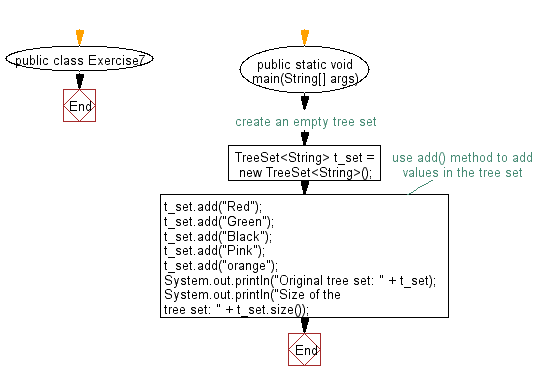﻿ Java: Get the number of elements in a tree set# Java Collection, TreeSet Exercises: Get the number of elements in a tree set

## Java Collection, TreeSet Exercises: Exercise-7 with Solution

Write a Java program to get the number of elements in a tree set.

Sample Solution:

Java Code:

``````import java.util.TreeSet;
import java.util.Iterator;

public class Exercise7 {
public static void main(String[] args) {
// create an empty tree set
TreeSet<String> t_set = new TreeSet<String>();
System.out.println("Original tree set: " + t_set);
System.out.println("Size of the tree set: " + t_set.size());
}
}
``````

Sample Output:

```Original tree set: [Black, Green, Pink, Red, orange]
Size of the tree set: 5 ```

Flowchart:Java Code Editor:

What is the difficulty level of this exercise?

Test your Programming skills with w3resource's quiz.

﻿

## Java: Tips of the Day

getEnumMap

Converts to enum to Map where key is the name and value is Enum itself.

```public static <E extends Enum<E>> Map<String, E> getEnumMap(final Class<E> enumClass) {
return Arrays.stream(enumClass.getEnumConstants())
.collect(Collectors.toMap(Enum::name, Function.identity()));
}
```

Ref: https://bit.ly/3xXcFZt